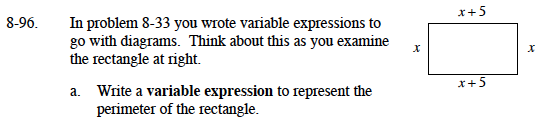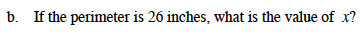### Home > MC1 > Chapter 8 > Lesson 8.3.2 > Problem8-96

8-96.Remember that the perimeter is the distance around the rectangle.

x + (x + 5) + x + (x + 5)Can you find the value of x that makes the perimeter expression you wrote equal to 26?